# Average monthly salary

A total of 10 teachers work at one small school in Moravia. The monthly salary of each is 21,500 CZK or 21,800 CZK or 22,500 CZK according to their education and age. The average monthly salary for this school's teacher is 21 850 CZK. How many teachers of this school earn CZK 22 5000 per month?

Correct result:

c =  2

#### Solution: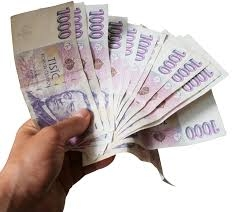We would be pleased if you find an error in the word problem, spelling mistakes, or inaccuracies and send it to us. Thank you!Tips to related online calculators
Looking for help with calculating arithmetic mean?
Looking for a statistical calculator?
Do you have a linear equation or system of equations and looking for its solution? Or do you have quadratic equation?
Do you solve Diofant problems and looking for a calculator of Diofant integer equations?

#### You need to know the following knowledge to solve this word math problem:

We encourage you to watch this tutorial video on this math problem:

## Next similar math problems:

• Bikes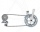Pepa asked Honza how many bikes he had. He replied that he had three-quarters of their total number and another three-quarter of one bike. So how many bikes does Honza have?
• Three workshopsThere are 2743 people working in three workshops. In the second workshop works 140 people more than in the first and in third works 4.2 times more than the second one. How many people work in each workshop?
• An animal shelter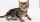An animal shelter spends $5.50 per day to care for each bird and$8.50 per day to care for each cat. Nicole noticed that the shelter spent $283.00 caring for birds and cats on Wednesday. Nicole found a record showing that there were a total of 40 birds an • Large familyI have as many brothers as sisters and each my brother has twice as many sisters as brothers. How many children do parents have? • At theAt the presentation of the travelers came three times as many men than women. When eight men left with their partners, there were five times more men than women at the presentation. How many were men and women originally? • 925 USDFour classmates saved an annual total 925 USD. The second save twice as the first, third 35 USD more than the second and fourth 10 USD less than the first. How USD save each of them? • Circus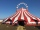On the circus performance was 150 people. Men were 10 less than women and children 50 more than adults. How many children were in the circus? • Stones 3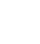Simiyu and Nasike each collected a number of stones in an arithmetic lesson. If Simiyu gave Nasike 5 stones, Nasike would have twice as many stones as Simiyu. If initially, Simiyu had five stones less than Nasike how many stones did each have? • Pears and orangesThere are 3 times as many pears as oranges. If a group of children receives 5 oranges each, there will be no oranges left over. If the same group of children receives 8 pears each, there will be 21 pears leftover. How many children and oranges are there? • Nine booksNine books are to be bought by a student. Art books cost$6.00 each and biology books cost $6.50 each . If the total amount spent was$56.00, how many of each book was bought?
• AverageIf the average(arithmetic mean) of three numbers x,y,z is 50. What is the average of there numbers (3x +10), (3y +10), (3z+10) ?
• SavingsPaul has a by half greater savings than half Stanley, but the same savings as Radek. Staney save 120 CZK less than Radek. What savings have 3 boys together?
• Three unknownsSolve the system of linear equations with three unknowns: A + B + C = 14 B - A - C = 4 2A - B + C = 0
• Ball gameRichard, Denis and Denise together scored 932 goals. Denis scored 4 goals over Denise but Denis scored 24 goals less than Richard. Determine the number of goals for each player.
• The dataThe data set represents the number of cars in a town given a speeding ticket each day for 10 days. 2 4 5 5 7 7 8 8 8 12 What is the IQR?
• Sum of two primesChristian Goldbach, a mathematician, found out that every even number greater than 2 can be expressed as a sum of two prime numbers. Write or express 2018 as a sum of two prime numbers.
• Linsys2Solve two equations with two unknowns: 400x+120y=147.2 350x+200y=144